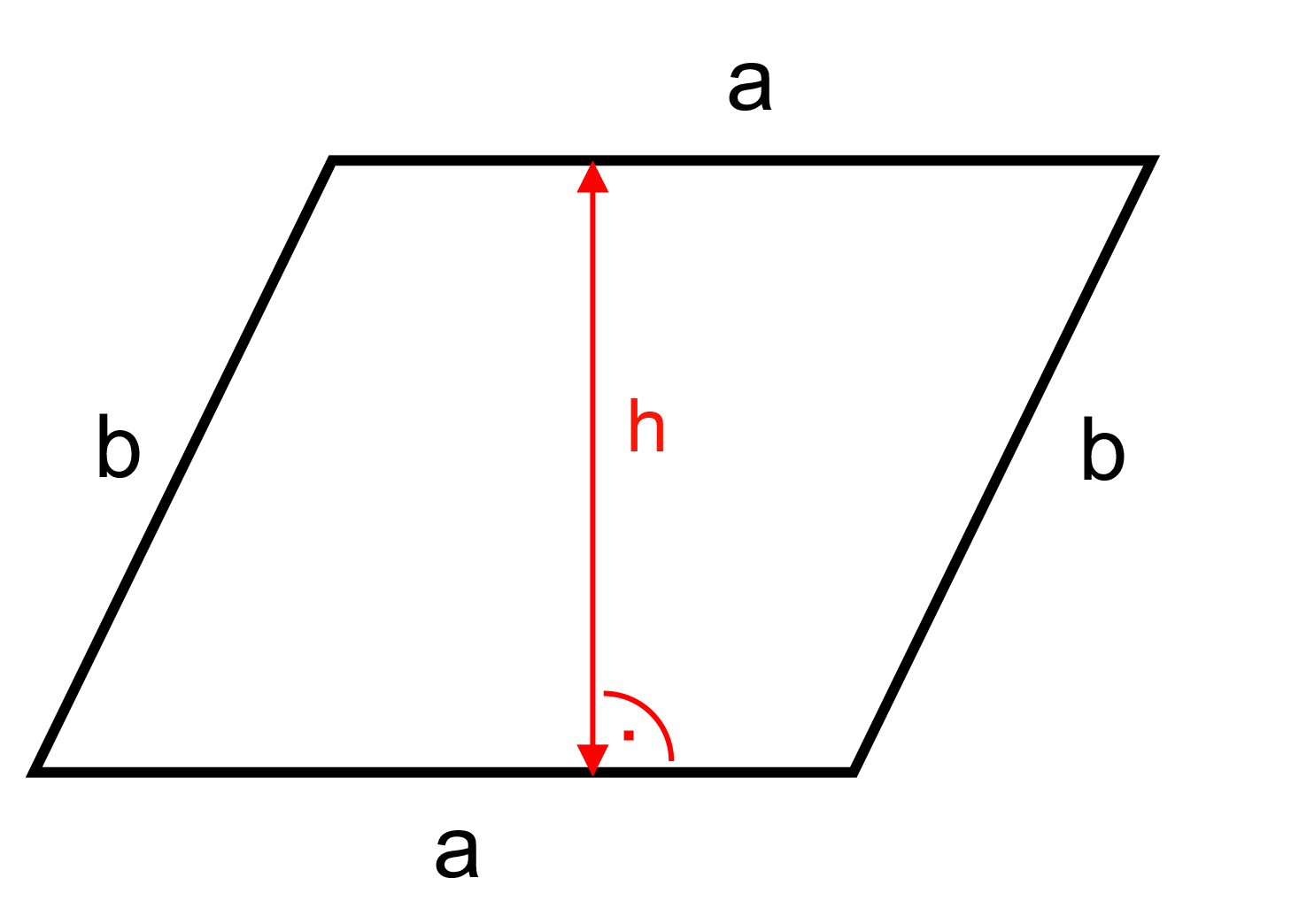﻿ Parallelogram: - on line calculation, formula - FORMIAX

# Parallelogram - circumference and surfaceC = 2 * a + 2 * b

S = a * v

C = circumference

S = surface area

a = side "a"

b = side "b"

h = height

WHAT IS IT ?
A 4-sided flat shape with straight sides where opposite sides are parallel.And
• opposite sides are equal in length, and
• opposite angles are equal

CALCULATION:

Enter unit e.g.: inch

Enter side "a"

Enter side "b"

Enter height "h"

Round to number of decimals places

YOU MIGHT BE INTERESTED: